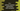# setbase operator in C++## C++ setbase function :

setbase() is a manipulator function. This is used to change the basefield of a value. This function is defined in iomanip library. So, we need to include it to use setbase.

### Function definition :

setbase is defined as below :

``setbase(int base)``

The parameter base can be either 10, 16 or 8. 10 is for decimal, 16 is for hexadecimal and 8 is for octal. If you pass any other value, it will be considered as zero. Note that we can also use hex, dec and oct to do the manipulation.

### C++ Example of setbase() function :

``````#include <iostream>
#include <iomanip>
using namespace std;
int main()
{
int n = 100;
cout << "Given number : " << n << endl;
cout << setbase(16);
cout << "Hexadecimal : " << n << endl;
}``````

This example program will print the below output :

``````Given number : 100

Here, we are using setbase to change it to hexadecimal mode. So, the last cout converted the value of n to hexadecimal.

### C++ example using dec, hex and oct :

``````#include <iostream>
#include <iomanip>
using namespace std;
int main()
{
int n = 100;
cout << "Hexadecimal : " << hex << n << endl;
cout << "Octal : " << oct << n << endl;
cout << "Decimal : " << dec << n << endl;
}``````

Here, we are using the keywords to do the conversion. It will print :

``````Hexadecimal : 64
Octal : 144
Decimal : 100``````

### C++ example for conversion using setbase :

Similar to the keywords we have used in the above example, we can also use setbase to manipulate the cout :

``````#include <iostream>
#include <iomanip>
using namespace std;
int main()
{
int n = 100;
cout << "Hexadecimal : " << setbase(16) << n << endl;
cout << "Octal : " << setbase(8) << n << endl;
cout << "Decimal : " << setbase(10) << n << endl;
}``````

The output will be the same as the above example.